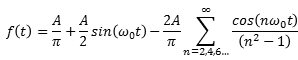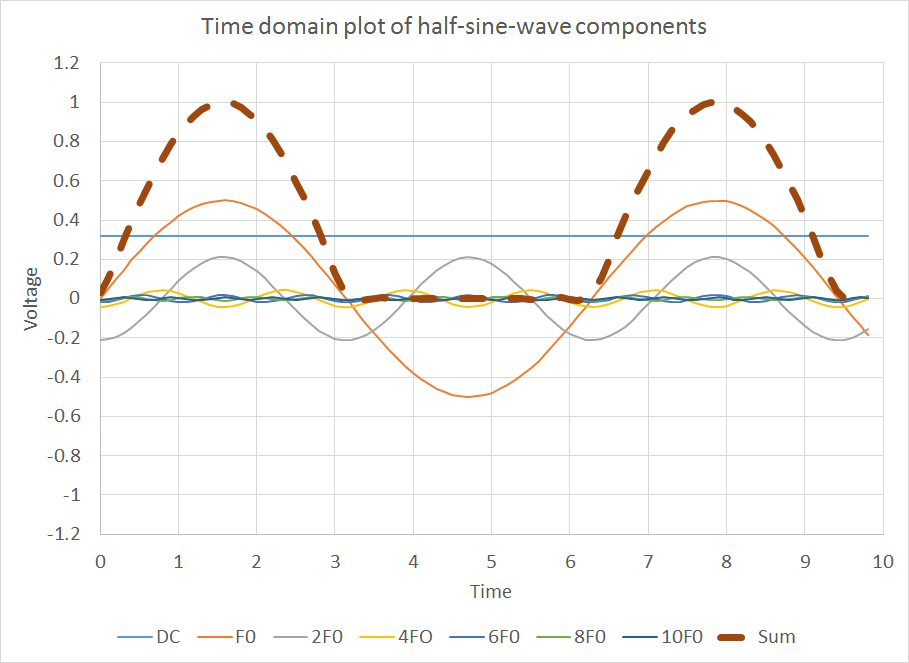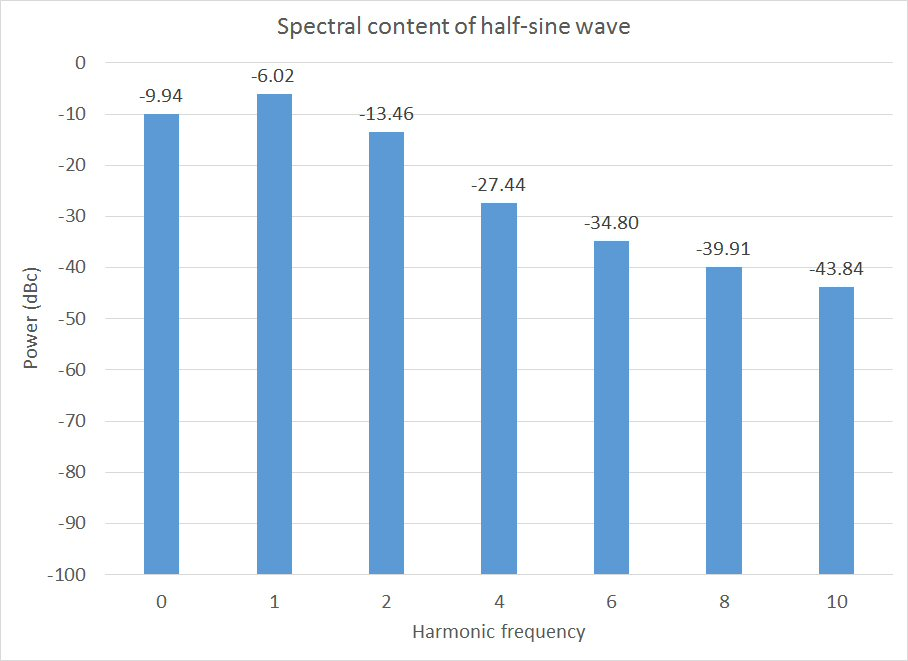# Fourier transforms

New for April 2016!

Perhaps no other field of engineering uses Fourier analysis as much as microwave engineering. Fourier transforms allow you to go back and forth between time and frequency domains. On this page we will present an example waveform, a half-sine wave. Joseph Fourier appears in the Microwaves101 Hall of Fame!

Fourier transforms of regular waveforms can be found in textbooks.  Arbitrary waveforms are best solved with FFT, or fast-Fourier transform data analysis.  You can perform this function in Matlab, or, believe it or not, in Microsoft Excel.  We'll save FFT for another day.

The Fourier series breaks down a waveform that is a function of time, to a series of sine waves at harmonic frequqnies and a series of cosine functions at harmonic frequencies.

## Even and odd functions

This is one of the greatest math shortcuts of all times...

Functions are said to be even, if f(t) always equals f(-t). The cosine function is an even function. For even functions, the b coefficients are zero.

Functions are said to be odd, if f(t) always equals -f(-t). The sine function is an odd function. For odd functions, the a coefficients are zero.

Look here for a better explanation.

## Fourier analysis of a half-sine wave

The Fourier series for a half-sine wave and other simple waveforms can be found in hundreds of textbooks and thousands of web sites.  You can even find it on RF Cafe! Here it is.  Three things are worth pointing out: the dirst term is the "DC" voltage. The second term is the only sine term in the series. The third term (cosine terms) has only even harmonics.We used Excel to calculate the the frequency coefficients to n=10, the tenth harmonic, then summed up the individual waves to see how well the half-sine is represented. The answer is, pretty well! The dotted lineis what you would see if you added a low pass filter at 11 GHz to a 1 GHz half-sine wave, and displayed the wave on a very expensive oscilloscope.Next, we looked at the amplitudes in the frequency domain.  This is exactly what you would expect if you displayed a half-sine wave on a spectrum analyzer. The baseband signal strength is down by 6 dB, compared to a full sine wave. If you added up all the power that is scattered around the spectrum, you'd see approximately 40% of the power contained in a full sine wave.